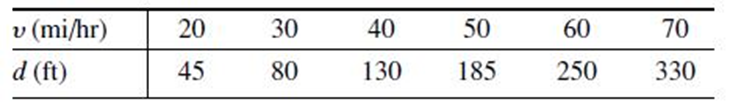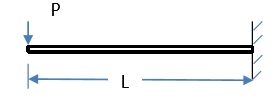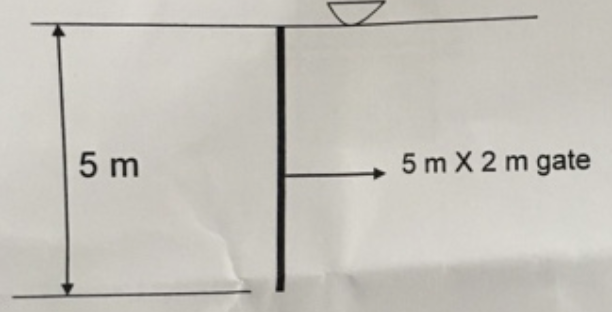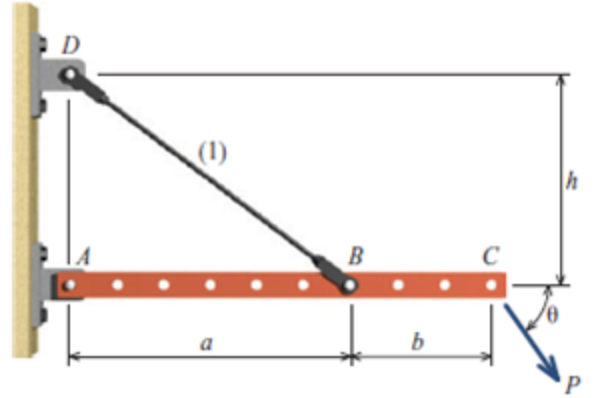##### The following data give the stopping distance d as a function of initial speed υ, for a certain

The following data give the stopping distance d as a function of initial speed υ, for a certain car model. Find a quadratic polynomial that fits the data. Determine the quality of the curve t by computing J, S, and r2.In: Mechanical Engineering

##### Suppose that z = xy, where x and y are independent and normally distributed random variables

Suppose that z = xy, where x and y are independent and normally distributed random variables. The mean and variance of x are µx = 10 and σ2x = 2. The mean and variance of y are µy = 15 and σ2y = 3. Find the mean and variance of z by simulation. Does µz = µxµy? Does σ2z = σ2x σ2y? Do this for 100, 1000, and 5000 trials.

In: Mechanical Engineering

##### Suppose that y = x2, where x is a normally distributed random variable with a mean

Suppose that y = x2, where x is a normally distributed random variable with a mean and variance of µx = 0 and σ2x = 4. Find the mean and variance of y by simulation. Does µy = µ2x? Does σy = σ2x? Do this for 100, 1000, and 5000 trials.

In: Mechanical Engineering

##### Measurements of a number of fittings show that the pitch diameter of the thread is normally

Measurements of a number of fittings show that the pitch diameter of the thread is normally distributed with a mean of 8.007 mm and a standard deviation of 0.005 mm. The design specifications require that the pitch diameter be 8 ± 0.01 mm. Estimate the percentage of fittings that will be within tolerance.

In: Mechanical Engineering

##### Data analysis of the breaking strength of a certain fabric shows that it is normally

Data analysis of the breaking strength of a certain fabric shows that it is normally distributed with a mean of 300 lb and a variance of 9.

a. Estimate the percentage of fabric samples that will have a breaking strength no less than 294 lb.

b. Estimate the percentage of fabric samples that will have a breaking strength no less than 297 lb and no greater than 303 lb.

In: Mechanical Engineering

##### Use the switch structure to write a MATLAB program to compute the amount of money that accumulates

Use the switch structure to write a MATLAB program to compute the amount of money that accumulates in a savings account in one year. The program should accept the following input: the initial amount of money deposited in the account; the frequency of interest compounding (monthly, quarterly, semiannually, or annually); and the interest rate. Run your program for a \$1000 initial deposit for each case; use a 5 percent interest rate. Compare the amounts of money that accumulate for each case.

In: Mechanical Engineering

##### A liquid (ρ = 1000 kg/m3; μ = 2 × 10–2 N ∙ s/m2; v = 2 × 10–5 m2/s) flows tangentially p

A liquid (ρ = 1000 kg/m3; μ = 2 × 10–2 N ∙ s/m2; v = 2 × 10–5 m2/s) flows tangentially past a flat plate with total length of 4 m (parallel to the flow direction), a velocity of 1 m/s, and a width of 1.5 m. What is the skin friction drag (shear force) on one side of the plate?

In: Mechanical Engineering

##### project planning

what is a critical path in project planning? give a brief explanation on how to draw the critical path diagram

In: Mechanical Engineering

##### Consider steam in an ideal Rankine cycle. The saturated vapor enters the turbine at 8.0 MPa.

Consider steam in an ideal Rankine cycle. The saturated vapor enters the turbine at 8.0 MPa. Saturated liquid exits the condenser at P = 0.008 MPa. The net power output of the cycle is 100 MW.

determine the thermal efficiency of the cycle

In: Mechanical Engineering

##### A gas-turbine power plant operates on the simple Brayton cycle between the pressure limits of 100 and 1200 kPa.

A gas-turbine power plant operates on the simple Brayton cycle between the pressure limits of 100 and 1200 kPa. The working ﬂuid is air, which enters the compressor at 300K at a rate of 150 m3/min and leaves the turbine at 773K. Using variable speciﬁc heats for air and assuming a compressor isentropic eﬃciency of 82 percent and a turbine isentropic eﬃciency of 88 percent, determine:

(a) the net power output

(b) the back work ratio

(c) the thermal eﬃciency

In: Mechanical Engineering

##### ericsson cycle

Consider an ideal Ericsson cycle with air as the working ﬂuid executed in a steady-ﬂow system. Air is at a temperature of 270 degrees and a pressure of  120 kPa at the beginning of the isothermal compression process, during which 150 kJ/kg of heat is rejected. Heat transfer to air occurs at a temperature of 1200K. Determine

(a) the maximum pressure in the cycle

(b) the network output per unit mass of air

(c) the thermal eﬃciency of the cycle

In: Mechanical Engineering

##### DEFLECTION OF BEAMS

Determine the maximum deflection on the Cantilever beam with a concentrated load p at the free end as shownIn: Mechanical Engineering

##### Printed on the side of a tire on an all-wheel-drive sport utility wagon is the warning “Do not inflate above 44 psi,

Printed on the side of a tire on an all-wheel-drive sport utility wagon is the warning “Do not inflate above 44 psi,” where psi is the abbreviation for the pressure unit pounds per square inch (lb/in2). Express the tire’s maximum pressure rating in

(a) The USCS unit of lb/ft2 (psf) and

(b) the SI unit of kPa.

In: Mechanical Engineering

##### (a) How do you calculate hydrostatic force in gates?

(a) How do you calculate hydrostatic force in gates?

(b) Calculate total hydrostatic force on the vertical gate. Also calculate the location of the hydrostatic force from topIn: Mechanical Engineering

##### Rigid bar ABC shown in Figure P1.30 is supported by a pin at bracket A and by tie rod (1).

Rigid bar ABC shown in Figure P1.30 is supported by a pin at bracket A and by tie rod (1). Tie rod (1) has a diameter of 5 mm, and it is supported by double-shear pin connections at B and D. The pin at bracket A is a single-shear connection. All pins are 7 mm in diameter. Assume a = 600 mm, b = 300 mm, h = 450 mm, P = 900 N, and θ = 55°. Determine the following:

(a) the normal stress in rod (1)

(b) the shear stress in pin B

(c) the shear stress in pin A

FIGURE P1.30In: Mechanical Engineering